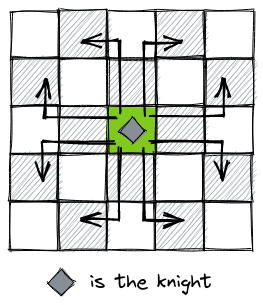# K for KnightAMDAD_MBSTUReplay of MBSTU CSE Junio...
Limits 1s, 512 MB

Alice is a famous chess player. Her friend Bob wants to learn chess. So she gives him a task. The task is Alice will give him a chess board with $n$ rows and $m$ columns. There will be one very special knight in a cell $(X,Y)$ on the board. Here, $X$ is the row number and $Y$ is the column number. That knight is special because Alice decides how it will move. She will set two integers $P$ and $Q$. The knight will be able to move $P$ squares by row (left or right) then $Q$ squares by column (up or down) or $P$ squares by column (up or down) then $Q$ squares by row (left or right).

Knight can not move outside of chessboard.

There are some cells in the board which are restricted, meaning knight can not move to those cells. Now he have to find the number of different cell that the knight can visit in a single move inside the board. Rows are numbered from 1 to $n$ and columns are numbered from 1 to $m$ and top-leftmost cell is $(1,1)$.The picture shows all possible moves of a knight in a $5 \times 5$ chess board from cell $(X=3,Y=3)$ and $(P=2,Q=1)$.

No cell is restricted in the above picture.

## Input

The first line of the input contains two integers $n$ and $m$ $(1 \leq n,m \leq 100)$. $n$ represents number of rows and $m$ represents number of columns of the chess board.

Second line of the input contains two integer $X$ and $Y$. $(X,Y)$ represents the initial position of the Knight. Here $X$ is the row number and $Y$ is the column number.

Third line contains $(P,Q)$. Details about $(P,Q)$ described in the statement.

Fourth line contains integer $Z(0 \leq Z \leq 10)$,number of restricted cells.

The $i-th$ of the next $Z$ lines contains two integers $C_i(1 \leq C_i \leq n)$ and $D_i (1 \leq D_i \leq m)$ — the position of the $i-th$ restricted cell.

$(1 \leq X \leq n)$ and $(1 \leq Y \leq m)$.

$(1 \leq P \leq 10)$ and $(1 \leq Q \leq10)$.

The initial position of the knight is not restricted.

## Output

Print the number of different cells the knight can visit in a single move.

## Samples

InputOutput
5 5
3 2
2 2
1
1 4

1

InputOutput
3 4
2 2
1 1
1
1 3

3


### Submit